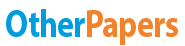# 1st Order Low Pass Filter Lab

Essay by   •  September 18, 2011  •  Case Study  •  1,376 Words (6 Pages)  •  2,036 Views

## Essay Preview: 1st Order Low Pass Filter Lab

Report this essay
Page 1 of 6

INTRODUCTION

The objective of this experiment is to design a passive, 1st order, low pass filter with a break frequency of 100 Hz. Following proper lab procedure the circuit will first be designed theoretically by developing the transfer function and using mathematical equations to arrive at a solution. The design will then be tested using both Excel and MultiSIM to verify the theoretical solution. Once the design is verified using MultiSIM and Excel, the physical construction of the circuit will be performed using National Instruments NI ELVIS and an analysis of its operation will be verified and recorded.

Theoretical Solution

Capacitive low pass filters work because the reactance of the capacitor is high at low frequencies and blocks any current flow through the capacitor effectively passing it all out the output. All frequencies that are below the designed cut off (break frequency) of 100Hz are passed with very little attenuation. The break frequency of 100Hz is the frequency at which the capacitive reactance and resistance become equal. At this point the output signal is attenuated to .707 volts of the one volt input (70.7 percent of any input). As the frequency continues to increase there is a roll off of -20dB/decade. The signals above 100Hz become greatly attenuated, until the reactance of the capacitor becomes so low that it acts like a short, resulting in zero output. Since the circuit contains a capacitor, the phase angle lags and at the frequency break (100Hz) it is out of phase by -45 degrees. This phase shift is caused by the time it takes the capacitor to charge and the higher the frequency the more it lags and becomes out of phase.

Figure 1

Results

Procedure:

The design of the 1st order, low pass filter with a break frequency of 100Hz will be done using mathematics. First a value for capacitance will be decided. Once the value for capacitance is decided and the value for break frequency is known, the value for resistance can be solved. In a 1st order low pass filter only two components are needed, theoretically speaking. Since it is a low pass filter, the resister is placed in the circuit first making it and the capacitor voltage in (Vin). The capacitor is placed second, making it voltage out (Vout). Refer to diagram one.

Diagram 1 Using mathematics, first the transfer function will be developed (refer to figure 1 equation 1.1 and 1.2). Having picked a capacitive value and already knowing the frequency at which the filter is designed to start attenuating, the frequency break formula can then algebraically be rearranged to find the resistance needed for the filter to perform as desired(refer to figure 1, equation 1.5). Once the filter has been theoretically designed, it will be tested it using MultiSIM (circuit simulation software). When testing with MultiSIM, the Bode Plotter will be used to check the filter is at 100Hz at -3dB down. The data was confirmed to be 100 percent accurate. Refer to diagram two.

Diagram 2

The next test performed using MultiSIM was to make sure the phase angle was lagging by -45 degrees at the break frequency. The delta time is divided by ten then multiplied by 360. Refer to diagram three.

Diagram 3

The next check used in this experiment was Windows Excel 2007. All calculations were imputed into Excel and graphed. Refer to diagram four.

Diagram 4

The circuit is now physically built and tested on the National Instruments NI ELVIS serial#126A01F. Due to the stock supply of components, three resistors and three capacitors were needed to make up the values needed per the design. Since three capacitors were needed for one value of capacitance they had to be put in parallel to combine the total values into one value. Capacitors in parallel add while capacitors in series divide (product over sum). Refer to diagram 5.

Diagram 5

...

...

Download as:   txt (7.2 Kb)   pdf (100.5 Kb)   docx (11.5 Kb)
Continue for 5 more pages »
Only available on OtherPapers.com
Citation Generator

(2011, 09). 1st Order Low Pass Filter Lab. OtherPapers.com. Retrieved 09, 2011, from https://www.otherpapers.com/essay/1st-Order-Low-Pass-Filter-Lab/11477.html

"1st Order Low Pass Filter Lab" OtherPapers.com. 09 2011. 2011. 09 2011 <https://www.otherpapers.com/essay/1st-Order-Low-Pass-Filter-Lab/11477.html>.

"1st Order Low Pass Filter Lab." OtherPapers.com. OtherPapers.com, 09 2011. Web. 09 2011. <https://www.otherpapers.com/essay/1st-Order-Low-Pass-Filter-Lab/11477.html>.

"1st Order Low Pass Filter Lab." OtherPapers.com. 09, 2011. Accessed 09, 2011. https://www.otherpapers.com/essay/1st-Order-Low-Pass-Filter-Lab/11477.html.